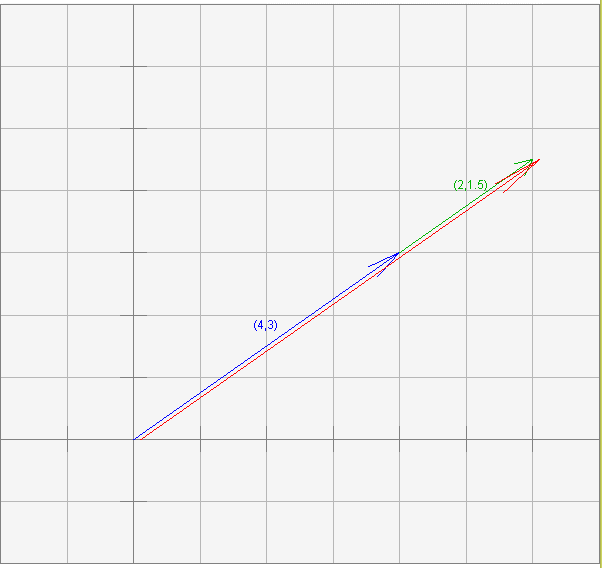This is true if one vector has the same direction as the other.

# Vectors in the Same DirectionThis situation is illustrated in the diagram. The head-to-tail rule is used to sum two vectors pointing in the same direction. (Vectors in the same direction are called collinear). The sum vector is slightly moved out of its correct position so that you can see it.

The summed vectors are ( 4, 3 )T and ( 2, 1.5 )T. The sum is (6, 4.5)T

For now, to determine if two vectors have the same direction look at a picture or use geometry. Later on you will see a procedure to test if two vectors point in the same direction.

This example is a case where the "=" sign in the formula applies:

```length(u + v) <= length(u) + length(v)
```

### QUESTION 18:

Can you think of another case in which the "=" sign applies?